• 参考文献：刘文臻. 中文文本多标签分类算法研究[D].电子科技大学,2020.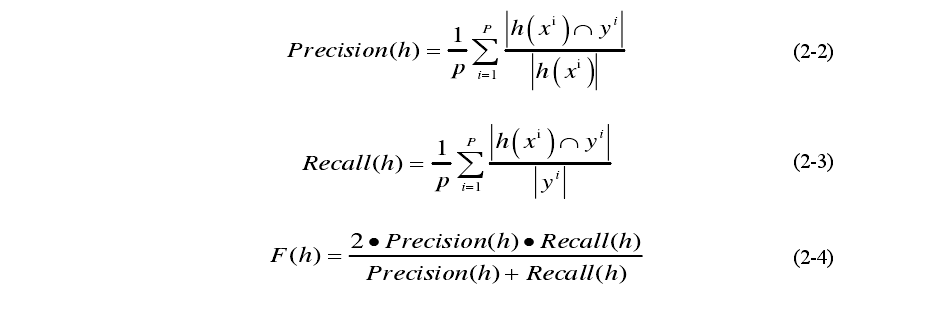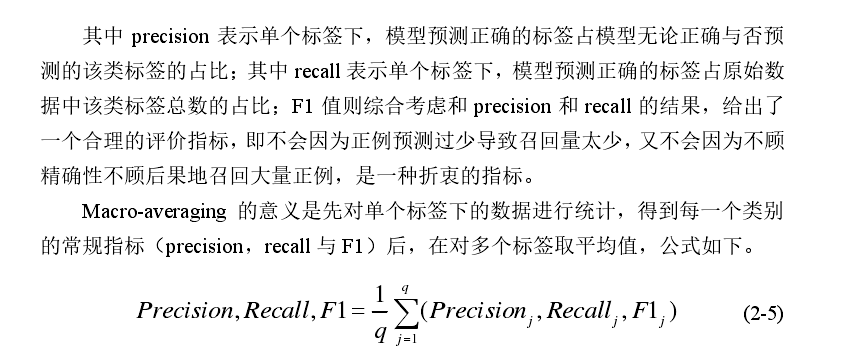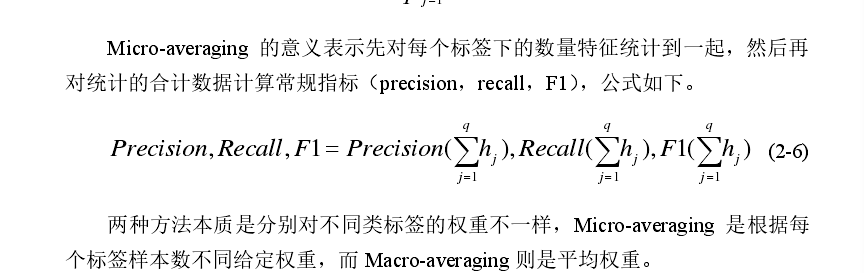参考文献：刘文臻. 中文文本多标签分类算法研究[D].电子科技大学,2020.
展开全文自然语言处理
•micro macro
• F1 score 召回率 recall ： 真实正例中预测为正的比例。 准确率 precision ： 预测为正的实例真实也为正（预测正确）的比例。 ROC曲线 其他展示分类模型性能的可视化技术是受试者特征曲线（ROC 曲线，Receiver ...
F1 score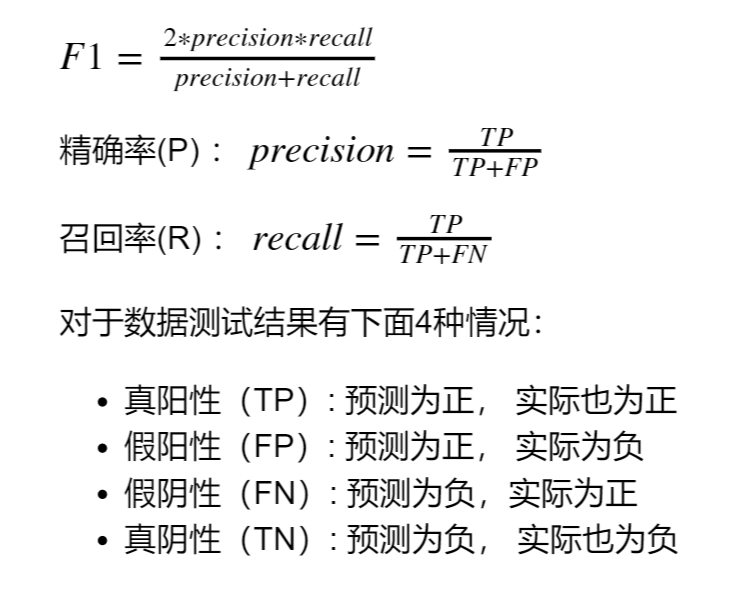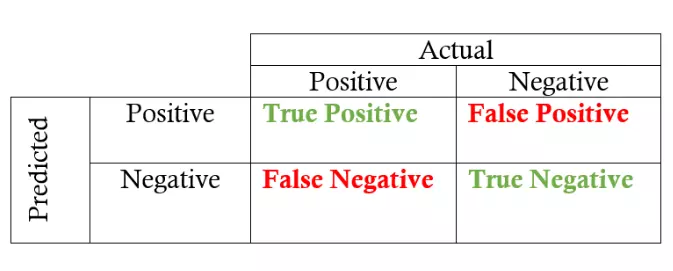召回率 recall ： 真实正例中预测为正的比例。 准确率 precision ： 预测为正的实例真实也为正（预测正确）的比例。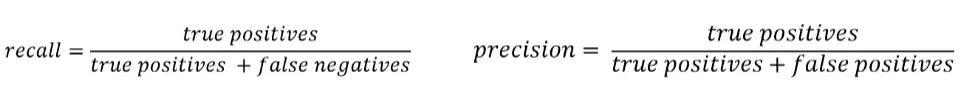ROC曲线 其他展示分类模型性能的可视化技术是受试者特征曲线（ROC 曲线，Receiver Operating Characteristic curve）。 这个思想是相当简单的：ROC 曲线展示了 当改变在模型**（预测）中识别为正例的阈值时，召回率和精确度的关系会如何变化。 ROC 曲线在 Y 轴上画出了真正例率（T
展开全文• 多标签分类 评价指标Metrics play quite an important role in the field of Machine Learning or Deep Learning. We start the problems with metric selection as to know the baseline score of a particular ...

多标签分类 评价指标

Metrics play quite an important role in the field of Machine Learning or Deep Learning. We start the problems with metric selection as to know the baseline score of a particular model. In this blog, we look into the best and most common metrics for Multi-Label Classification and how they differ from the usual metrics.
指标在机器学习或深度学习领域中扮演着非常重要的角色。 我们从度量选择开始着手，以了解特定模型的基线得分。 在此博客中，我们研究了“多标签分类”的最佳和最常用指标，以及它们与通常的指标有何不同。
Let me get into what is Multi-Label Classification just in case you need it. If we have data about the features of a dog and we had to predict which breed and pet category it belonged to.
让我进入什么是多标签分类，以防万一您需要它。 如果我们有关于狗的特征的数据，并且我们必须预测它属于哪个品种和宠物。
In the case of Object Detection, Multi-Label Classification gives us the list of all the objects in the image as follows. We can see that the classifier detects 3 objects in the image. It can be made into a list as follows [1 0 1 1] if the total number of trained objects are 4 ie. [dog, human, bicycle, truck].
在对象检测的情况下，多标签分类为我们提供了图像中所有对象的列表，如下所示。 我们可以看到分类器检测到图像中的3个对象。 如果训练对象的总数为4，即[1 0 1 1]，则可以将其列为以下列表。 [狗，人，自行车，卡车]。
Object Detection output

This kind of classification is known as Multi-Label Classification.
这种分类称为多标签分类。
The most common metrics that are used for Multi-Label Classification are as follows:
用于多标签分类的最常见指标如下：
Precision at k k精度 Avg precision at k平均精度(k)Mean avg precision at k k的平均平均精度 Sampled F1 Score采样的F1分数
Let’s get into the details of these metrics.
让我们详细了解这些指标。
k精度(P @ k)： (Precision at k (P@k):)
Given a list of actual classes and predicted classes, precision at k would be defined as the number of correct predictions considering only the top k elements of each class divided by k. The values range between 0 and 1.
给定实际类别和预测类别的列表，将在k处的精度定义为仅考虑每个类别的前k个元素除以k得出的正确预测数。 取值范围是0到1。
Here is an example as explaining the same in code:
这是一个解释相同代码的示例：
def patk(actual, pred, k):
#we return 0 if k is 0 because
#   we can't divide the no of common values by 0
if k == 0:
return 0

#taking only the top k predictions in a class
k_pred = pred[:k]

#taking the set of the actual values
actual_set = set(actual)

#taking the set of the predicted values
pred_set = set(k_pred)

#taking the intersection of the actual set and the pred set
# to find the common values
common_values = actual_set.intersection(pred_set)

return len(common_values)/len(pred[:k])

#defining the values of the actual and the predicted class
y_true = [1 ,2, 0]
y_pred = [1, 1, 0]

if __name__ == "__main__":
print(patk(y_true, y_pred,3))
Running the following code, we get the following result.
运行以下代码，我们得到以下结果。
0.6666666666666666
In this case, we got the value of 2 as 1, thus resulting in the score going down.
在这种情况下，我们将2的值设为1，从而导致得分下降。
K处的平均精度(AP @ k)： (Average Precision at K (AP@k):)
It is defined as the average of all the precision at k for k =1 to k. To make it more clear let’s look at some code. The values range between 0 and 1.
它定义为k = 1至k时k处所有精度的平均值。 为了更加清楚，让我们看一些代码。 取值范围是0到1。
import numpy as np
import pk

def apatk(acutal, pred, k):
#creating a list for storing the values of precision for each k
precision_ = []
for i in range(1, k+1):
#calculating the precision at different values of k
#      and appending them to the list
precision_.append(pk.patk(acutal, pred, i))

#return 0 if there are no values in the list
if len(precision_) == 0:
return 0

#returning the average of all the precision values
return np.mean(precision_)

#defining the values of the actual and the predicted class
y_true = [[1,2,0,1], [0,4], , [1,2]]
y_pred = [[1,1,0,1], [1,4], , [1,3]]

if __name__ == "__main__":
for i in range(len(y_true)):
for j in range(1, 4):
print(
f"""
y_true = {y_true[i]}
y_pred = {y_pred[i]}
AP@{j} = {apatk(y_true[i], y_pred[i], k=j)}
"""
)
Here we check for the AP@k from 1 to 4. We get the following output.
在这里，我们检查从1到4的AP @ k。我们得到以下输出。
y_true = [1, 2, 0, 1]
y_pred = [1, 1, 0, 1]
AP@1 = 1.0

y_true = [1, 2, 0, 1]
y_pred = [1, 1, 0, 1]
AP@2 = 0.75

y_true = [1, 2, 0, 1]
y_pred = [1, 1, 0, 1]
AP@3 = 0.7222222222222222

y_true = [0, 4]
y_pred = [1, 4]
AP@1 = 0.0

y_true = [0, 4]
y_pred = [1, 4]
AP@2 = 0.25

y_true = [0, 4]
y_pred = [1, 4]
AP@3 = 0.3333333333333333

y_true = 
y_pred = 
AP@1 = 0.0

y_true = 
y_pred = 
AP@2 = 0.0

y_true = 
y_pred = 
AP@3 = 0.0

y_true = [1, 2]
y_pred = [1, 3]
AP@1 = 1.0

y_true = [1, 2]
y_pred = [1, 3]
AP@2 = 0.75

y_true = [1, 2]
y_pred = [1, 3]
AP@3 = 0.6666666666666666
This gives us a clear understanding of how the code works.
这使我们对代码的工作方式有了清晰的了解。
K处的平均平均精度(MAP @ k)： (Mean Average Precision at K (MAP@k):)
The average of all the values of AP@k over the whole training data is known as MAP@k. This helps us give an accurate representation of the accuracy of whole prediction data. Here is some code for the same.
整个训练数据中AP @ k所有值的平均值称为MAP @ k。 这有助于我们准确表示整个预测数据的准确性。 这是一些相同的代码。
The values range between 0 and 1.
取值范围是0到1。
import numpy as np
import apk

def mapk(acutal, pred, k):

#creating a list for storing the Average Precision Values
average_precision = []
#interating through the whole data and calculating the apk for each
for i in range(len(acutal)):
average_precision.append(apk.apatk(acutal[i], pred[i], k))

#returning the mean of all the data
return np.mean(average_precision)

#defining the values of the actual and the predicted class
y_true = [[1,2,0,1], [0,4], , [1,2]]
y_pred = [[1,1,0,1], [1,4], , [1,3]]

if __name__ == "__main__":
print(mapk(y_true, y_pred,3))
Running the above code, we get the output as follows.
运行上面的代码，我们得到的输出如下。
0.4305555555555556
Here, the score is bad as the prediction set has many errors.
在此，由于预测集存在许多错误，因此评分很差。
F1-样本： (F1 — Samples:)
This metric calculates the F1 score for each instance in the data and then calculates the average of the F1 scores. We will be using sklearn’s implementation of the same in the code.
此度量标准计算数据中每个实例的F1分数，然后计算F1分数的平均值。 我们将在代码中使用sklearn的相同实现。
Here is the documentation of F1 Scores. The values range between 0 and 1.
这是F1分数的文档。 取值范围是0到1。
We first convert the data into binary format and then perform f1 on the same. This gives us the required values.
我们首先将数据转换为二进制格式，然后对它执行f1。 这为我们提供了所需的值。
from sklearn.metrics import f1_score
from sklearn.preprocessing import MultiLabelBinarizer

def f1_sampled(actual, pred):
#converting the multi-label classification to a binary output
mlb = MultiLabelBinarizer()
actual = mlb.fit_transform(actual)
pred = mlb.fit_transform(pred)

#fitting the data for calculating the f1 score
f1 = f1_score(actual, pred, average = "samples")
return f1

#defining the values of the actual and the predicted class
y_true = [[1,2,0,1], [0,4], , [1,2]]
y_pred = [[1,1,0,1], [1,4], , [1,3]]

if __name__ == "__main__":
print(f1_sampled(y_true, y_pred))
The output of the following code will be the following:
以下代码的输出如下：
0.45
We know that the F1 score lies between 0 and 1 and here we got a score of 0.45. This is because the prediction set is bad. If we had a better prediction set, the value would be closer to 1.
我们知道F1分数介于0和1之间，在这里我们得到0.45的分数。 这是因为预测集不好。 如果我们有更好的预测集，则该值将接近1。
Hence based on the problem, we usually use Mean Average Precision at K or F1 Sample or Log Loss. Thus setting up the metrics for your problem.
因此，基于该问题，我们通常使用K或F1样本或对数损失的平均平均精度。 从而为您的问题设置指标。
I would like to thank Abhishek for his book Approaching (Any) Machine Learning Problem without which this blog wouldn’t have been possible.
我要感谢Abhishek的书《接近(任何)机器学习问题》，否则就没有这个博客。

翻译自: https://medium.com/analytics-vidhya/metrics-for-multi-label-classification-49cc5aeba1c3

多标签分类 评价指标

展开全文机器学习 python 人工智能 深度学习 大数据
• 对于多标签分类的评价指标比较复杂，一般对于n个二分类混淆矩阵要引入宏平均、微平均、权重评价即Macro-average、Micro-Average，Weighted-Average，近期正好应用，所以就总结一下。 Macro-averaging：指所有类别的...机器学习 评价指标
• 类分类与多标签分类 类分类(multi-class classification)：有个类别需要分类，但一个样本只属于一个类别 多标签分类(multi-label classificaton)：每个样本有个标签 区别： 对于类分类，最后一...深度学习
• 多标签分类作为分类的一种推广，每个样本可以有个类别，如下图的标签为：sea，sunset。所以其评价指标与分类的也有差异，本文将介绍几种评价指标。  1.Hamming loss(汉明损失)，表示所有label中错误样本...机器学习评价指标
• 多标签分类的算法，通常改变算法或数据，最常用的方法如下： 改造成二分类 比如，y标签一共有L个分类，那么就建立L个分类器，分别对应L个分类标签，进行训练。 标签排序+二分类 使用“成对比较”（pairwise ...
• 文本分类一般可以分为二分类、分类、多标签分类三种情况,二分类是指将一组文本分成两个类(0或1),比较常见的应用如垃圾邮件分类、电商网站的用户评价数据的正负面分类等,分类是指将文本分成若干个类中的某一个类,...分类算法评估
• 这是一个教程，说明了如何使用TensorFlow 2.0构建和训练用于多标签图像分类的机器学习系统。 例如，我们能否仅根据海报来预测电影的类型？ 我们将使用Kaggle上托管的电影海报数据集。 给定电影海报的图像，模型应...
• 分类和多标签分类的区别 分类： 一个样本属于且只属于个分类中的一个，一个样本只能属于一个类，不同类之间是互斥的，比如图片中一个狗只能被划分成狗，不能被划分成猫。 多标签分类： 一个样本可以属于个...深度学习 机器学习
• 一、多标签分类概述与总论 多标签分类，是一种有别于类分类的分类类型，举个例子: 假设有类["酸", "甜", "苦", "辣", "香"]， 类分类就是 "这个水果点酸"(label="酸")，"这个菜看起来很香"(label="香")； ...multi 短文本
• 本文将会介绍如何使用keras-bert实现文本多标签分类任务，其中对BERT进行微调。 项目结构 数据集介绍 模型训练 模型评估 模型预测 总结NLP
• keras提供了flow_from_directory用于单个标签分类，但是对图片的多标签分类没有支持，这就需要我们自己动手实现ImageDataGenerator,我这里把我实现的用于多标签分类的自定义DataGenerator分享出来，读者可以根据自己...
• TextCNN ...提取码：zygc TextCNN是卷积神经网络的一种(其实就是一个简单的神经网络)。 卷积神经网络是指-至少在网络的一层中使用了卷积运算代替矩阵乘法运算。...• 参数共享：个函数相同参数 • 等变表...自然语言处理 nlp 深度学习 机器学习
• 上个月和同事参加了Kaggle蛋白质图集多标签分类竞赛，获得第5，转发下同事写的Solution。 https://www.kaggle.com/c/human-protein-atlas-image-classification/discussion/77731 First of all, congratulations ...Kaggle
• import sklearn import numpy as np from scipy.sparse import csr_matrix from scipy.sparse.csgraph import laplacian from scipy.sparse.linalg...from sklearn.metrics import f1_score from sklearn.metrics impo.
• 网络上关于Precision、Recall和F1的介绍有很，因此这里只作简单回顾。 在二分类问题中，根据真实类别和预测类别的组合可以分为四中情况，分别是TP(True Positive)、FP(False Positive)、TN(True Negative)、FN...tensorflow 评估指标
• 本项目采用hamming loss对多标签分类进行评估，结果如下： precision recall f1-score support 0 1.0000 0.9167 0.9565 12 1 1.0000 0.1250 0.2222 8 2 1.0000 0.4000 0.5714 10 3 1.0000 0.6364 0.7778 11Python
• TP: 预测为正， 实现为正 FP: 预测为正， 实现为负 FN: 预测为负，实现为正 TN: 预测为负， 实现为负 准确率： TP/ (TP+FP) 召回率： TP(TP + FN) ...#class：分类标签 f1_fun = function(pre,y,class){ tp=NA...
• multi-label分类，常见一张图片中可以存在个目标，如猫，狗，人。这样这张图片就有三个标签； 2）multi-label分类和multi-class 分类？ multi-class 分类，就是将多种类别的图片进行类别分类，与multi-la...TensorRT
• 最近在做一个multilabel classification（多标签分类）的项目，需要一些特定的metrics去评判一个multilabel classifier的优劣。这里对用到的三个metrics做一个总结。 首先明确一下标签（multilabel）分类和类别...
• 本文同步发布知乎：HFT-CNN:层级多标签分类，让你的模型学习几次知乎主页lynne阿黎请大家不吝关注~ 背景 长文本由于自身的特点和信息量，一段文本会描述一个特定的主题。短文本由于其自身长度的原因是缺少这种...
• 基于标签图叠加的多标签分类 摘要   图像或视频总是包含个对象或动作。由于深度学习技术的快速发展，标签识别已经实现了良好的性能.近年来，图卷积网络(GCN)被用来提高标签识别的性能,然而，标签相关建模的...计算机视觉
• from sklearn.metrics import f1_score f1_score(y_train_5, y_train_pred)python
• 为了捕获和探索这种重要的依赖关系，我们提出了一种基于图卷积网络(Graph Convolutional Network GCN)的多标签分类模型。该模型在对象标签上构建了一个有向图，其中每个节点(标签)用标签的单词嵌入表示，GCN学习了...计算机视觉...